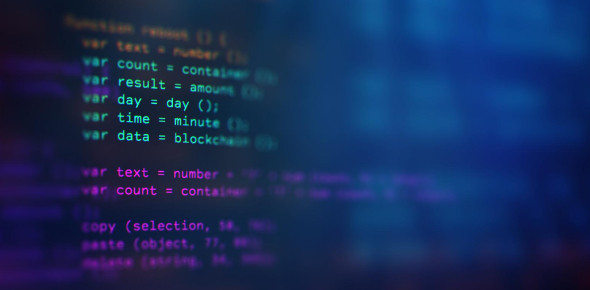# Arduino Quiz Questions And Answers

11 Questions | Attempts: 15378
ShareSettingsHere is an interesting quiz with informative questions and answers about Arduino and its related concepts. Arduino is an open-source hardware and software company, that deals in designing and manufacturing single-board microcontrollers and microcontroller kits for building digital devices. If you think you have good knowledge about Arduino, then you must take up this quiz and see how well you can score. So, are you ready? Let's start then. Good luck!

• 1.
What is the range of numbers you can store in a variable of type byte?
• A.

0 to 255

• B.

-128 to 127

• C.

0 to 9

• D.

0 to 65535

• E.

0 to 4294967295

• 2.
What does this line of code do? counter++;
• A.

Increment counter by an amount that depends on its definition.

• B.

This is not a legal statement in the Arduino language.

• C.

Adds 1 to the variable counter

• D.

Returns true if the variable counter has a positive value.

• E.

None of the above

• 3.
To subtract 1 from a variable counter, you can use the following statement(s) (check all that apply)
• A.

Counter - 1;

• B.

Counter--;

• C.

--counter;

• D.

Counter -= 1;

• E.

Decrement(counter);

• F.

Counter = counter - 1;

• 4.
What is the index of the letter F in this array?   char textMessage[] = "Have Fun with Arduino";
• A.

5

• B.

6

• C.

4

• D.

'F'

• E.

&textMessage+5

• 5.
How many elements are in the array?   char textMessage[] = "Have Fun with Arduino";
• A.

20

• B.

21

• C.

22

• D.

The statement is not a legal Arduino program statement.

• E.

None of the above

• 6.
Is there anything wrong with this line of code, if yes, what?   if(myVar = true) Serial.println("The light is ON");
• A.

The = should be ==

• B.

The line has invalid syntax, and will produce a compiler error.

• C.

There needs to be a space after the if keyword.

• D.

There is nothing wrong.

• E.

An if statement must use braces.

• 7.
The "Arduino Language" is best described as ... ?
• A.

A subset of standard C++

• B.

Similar to Java

• C.

A special purpose language designed for beginning programmers.

• D.

A subset of C#

• E.

• 8.
Which language features are NOT present in the normal Arduino environment? (Check all that apply)
• A.

Inheritance

• B.

Standard Template Library

• C.

Exceptions

• D.

• E.

Floating Point math operations

• F.

Object Oriented Programming

• G.

Pointers

• H.

Files

• 9.
What does the word static do in this line of code?   static int temperature = 0;
• A.

The variable's value is retained the next time it is accessed.

• B.

The value of temperature will not be allowed to change.

• C.

The variable will be reset to 0 each time loop() runs.

• D.

The variable will have a greater range than a normal int

• E.

The line is not a legal Arduino program statement.

• F.

None of the above

• 10.
What is the value of foo when these lines of code are executed?   foo = 12; foo = foo % 10;
• A.

1

• B.

2

• C.

1.2

• D.

None of the above

• 11.
What does this line of code do?   temp = !temp;
• A.

Sets the variable temp to its logical compliment.

• B.

Converts the variable temp to a boolean value.

• C.

The line is not a legal Arduino statement.

• D.

None of the above

• E.

Performs a factorial calculation.

## Related TopicsBack to top
×

Wait!
Here's an interesting quiz for you.